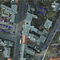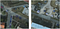# Giving SIMRDWN a Spin, Part I

## Training a model to detect cars in overhead images

The SIMRDWN framework extends popular object detection algorithms to operate in the overhead imagery domain. In brief, SIMRDWN extends the YOLT enhancements of YOLO to include the models of the TensorFlow Object Detection API. A previous post introduced SIMRDWN, and subsequent posts (1, 2) illustrated its application towards super-resolution techniques. In this post we demonstrate how to train a model from scratch with SIMRDWN.

We begin with one of the canonical use cases for satellite imagery analytics: car localization. We utilize the excellent COWC dataset, which contains 15 cm imagery and centroid labels for over 30,000 cars in six distinct locations. In the sections below we walk the reader through all the steps to train a model from scratch. The code to run all the commands detailed here can be found at the SIMRDWN github repository.

## 1. COWC Data Format

COWC labels consist of an image mask with non-zero pixels at the centroid of each car. We render these centroids into bounding boxes by assuming a median car size of 3 meters, or 20 pixels at 0.15 m resolution. Such an assumption works well for objects such as cars with a low variance in physical size (see Figure 1).Figure 1. COWC car labels (red dots) overlaid on an image over Potsdam. Inferred 3m bounding box labels for object detection are overlaid in blue.

## 2. Installation

We assume the reader has already installed SIMRDWN, (as described in the GitHub README) and has spun up a SIMRDWN docker container with a command such as:

`# path/to/simrdwn/ is the location of your local installnvidia-docker run -it -v /path/to/simrdwn:/path/to/simrdwn --name simrdwn_train simrdwn`

All commands should be run in this docker container.

## 3. Prepare YOLT training data

YOLO (and hence YOLT) requires training images to be located in an “images” folder and bounding box labels in a “labels” folder. For example, an image “images/ex0.png” has a corresponding label “labels/ex0.txt.” We also need to define the object classes with a .pbtxt file, such as /simrdwn/data/class_labels_car.pbtxt. Labels are bounding boxes of the form:

<object-class> <x> <y> <width> <height>

Where x, y, width, and height are fractions relative to the image’s width and height. Object-class is a zero-indexed integer.

The COWC images are far too large to train on natively, so must be sliced into smaller bins for training purposes. The slice_im_cowc() function in /simrdwn/core/parse_cowc.py creates training chips of the appropriate size, as well as creating the necessary directory structure, naming conventions, and coordinate transformations.

`def slice_im_cowc(input_im, input_mask, outname_root, outdir_im,                   outdir_label, classes_dic, category,                   yolt_box_size, sliceHeight=256,                   sliceWidth=256, zero_frac_thresh=0.2,                   overlap=0.2, pad=0, verbose=False,                  box_coords_dir='', yolt_coords_dir=''):    '''    Slice large satellite image into smaller pieces,     Ignore slices with a percentage null greater     than zero_frac_thresh. Assume input_im is rgb    '''    image = cv2.imread(input_im, 1)  # color    gt_image = cv2.imread(input_mask, 0)    category_num = classes_dic[category]        im_h, im_w = image.shape[:2]    win_size = sliceHeight*sliceWidth        # if slice sizes are large than image, pad the edges    if sliceHeight > im_h:        pad = sliceHeight - im_h    if sliceWidth > im_w:        pad = max(pad, sliceWidth - im_w)    # pad the edge of the image with black pixels    if pad > 0:            border_color = (0,0,0)        image = cv2.copyMakeBorder(image, pad, pad, pad, pad,                         cv2.BORDER_CONSTANT, value=border_color)    t0 = time.time()    n_ims = 0    n_ims_nonull = 0    dx = int((1. - overlap) * sliceWidth)    dy = int((1. - overlap) * sliceHeight)    for y in range(0, im_h, dy):        for x in range(0, im_w, dx):            n_ims += 1            # extract image            # make sure we don't go past the edge of the image            if y + sliceHeight > im_h:                y0 = im_h - sliceHeight            else:                y0 = y            if x + sliceWidth > im_w:                x0 = im_w - sliceWidth            else:                x0 = x                        window_c = image[y0:y0+sliceHeight, x0:x0+sliceWidth]            gt_c = gt_image[y0:y0+sliceHeight, x0:x0+sliceWidth]            win_h, win_w = window_c.shape[:2]                        # get black and white image            window = cv2.cvtColor(window_c, cv2.COLOR_BGR2GRAY)            # find threshold of image that's not black            ret,thresh1 = cv2.threshold(window, 2, 255,                                         cv2.THRESH_BINARY)            non_zero_counts = cv2.countNonZero(thresh1)            zero_counts = win_size - non_zero_counts            zero_frac = float(zero_counts) / win_size            # skip if image is mostly empty            if zero_frac >= zero_frac_thresh:                if verbose:                    print ("Zero frac too high at:", zero_frac)                continue                         box_coords, yolt_coords = \                        gt_boxes_from_cowc_png(gt_c,                                                yolt_box_size,                                                verbose=verbose)            # continue if no coords            if len(box_coords) == 0:                continue                        #  save                      outname_part = 'slice_' + outname_root \                + '_' + str(y0) + '_' + str(x0) + '_' \                + str(win_h) + '_' + str(win_w) \                + '_' + str(pad)            outname_im = os.path.join(outdir_im,                                       outname_part + '.png')            txt_outpath = os.path.join(outdir_label,                                        outname_part + '.txt')                        # save yolt ims            if verbose:                print ("image output:", outname_im)            cv2.imwrite(outname_im, window_c)                        # save yolt labels            txt_outfile = open(txt_outpath, "w")            if verbose:                print ("txt output:" + txt_outpath)            for bb in yolt_coords:                outstring = str(category_num) \                    + " " + " ".join([str(a) for a in bb]) + '\n'                if verbose:                    print ("outstring:", outstring)                txt_outfile.write(outstring)            txt_outfile.close()            n_ims_nonull += 1    print ("Num slices:", n_ims, "Num non-null slices:", \           n_ims_nonull, "sliceHeight", sliceHeight, "sliceWidth",             sliceWidth)    print ("Time to slice", input_im, time.time()-t0, "seconds")          return`

Detailed instructions for running the above code block can be found in the /simrdwn/core/prep_data_cowc.py script. Let’s plot a few bounding boxes to ensure they were created correctly, using the following function:

`yolt_data_prep_funcs.plot_training_bboxes(labels_dir, images_dir,      sample_label_vis_dir=sample_label_vis_dir)`Figure 2. Training image chips created by slice_im_cowc(), and visualized with plot_training_bboxes(). Bounding box labels overlaid in blue.

## 3. Create .tfrecord

The TensorFlow Object Detection API models require a .tfrecord data format for training. We can explicitly create a .tfrecord from the YOLT labels via the /simrdwn/core/preprocess_tfrecords.py script:

`def yolt_to_tf_example(image_file, label_file,                        label_map_dict,                       convert_dict={},                       ignore_difficult_instances=False,                       labelfile_columns=['cat_int',                                     'x_frac', 'y_frac',                                     'width_frac', 'height_frac'],                       verbose=False):  """  Create tfrecord from yolt image_flle and label_file  Adapted from:      https://github.com/tensorflow/models/blob/master/        research/object_detection/dataset_tools/        create_pascal_tf_record.py    convert_dict maps yolt internal labels to the      integers for .pbtxt   """      # read image file  im = cv2.imread(image_file, 1)  height, width = im.shape[:2]        with tf.gfile.GFile(image_file, 'rb') as fid:    encoded_jpg = fid.read()  key = hashlib.sha256(encoded_jpg).hexdigest()  xmin, ymin, xmax, ymax = [], [], [], []  classes, classes_text = [], []  if len(label_file) > 0:      # read label file      df = pd.read_csv(label_file, sep=' ', names=labelfile_columns)          for idx,row in df.iterrows():                cat_int, x_frac, y_frac, width_frac, height_frac = row        # get pixel coords        [x0, x1, y0, y1] = \            convert_bbox_yolt_to_tf(height, width, row)                   xmin.append(x0)         ymin.append(y0)        xmax.append(x1)         ymax.append(y1)         if len(convert_dict.keys()) > 0:            cat_int_out = convert_dict[cat_int]        else:            cat_int_out = cat_int        classes.append(int(cat_int_out))         classes_text.append(label_map_dict[cat_int_out])  example = tf.train.Example(features=tf.train.Features(    feature={      'image/height': int64_feature(height),      'image/width': int64_feature(width),      'image/filename': bytes_feature(          image_file.encode('utf8')),      'image/source_id': bytes_feature(          image_file.encode('utf8')),      'image/key/sha256': bytes_feature(key.encode('utf8')),      'image/encoded': bytes_feature(encoded_jpg),      'image/format': bytes_feature('jpeg'.encode('utf8')),      'image/object/bbox/xmin': float_list_feature(xmin),      'image/object/bbox/xmax': float_list_feature(xmax),      'image/object/bbox/ymin': float_list_feature(ymin),      'image/object/bbox/ymax': float_list_feature(ymax),      'image/object/class/text': bytes_list_feature(classes_text),      'image/object/class/label': int64_list_feature(classes),    }))        return example`

## 4. Training

We are now in a position to train an object detection model. We can use the YOLT architecture, or any architecture included in the TensorFlow Object Detection API. TensorFlow configs reside in the /simrdwn/configs directory, while YOLT configs can be found in /simrdwn/yolt/cfg. Execute training within the docker container with commands such as:

`# SSD COWC car searchpython /path/to/simrdwn/core/simrdwn.py \    --framework ssd \    --mode train \    --outname inception_v2_cowc \    --label_map_path /path/to/simrdwn/data/class_labels_car.pbtxt \    --tf_cfg_train_file /path/to/simrdwn/configs/_orig/ssd_inception_v2_simrdwn.config \    --train_tf_record /path/to/simrdwn/data/cowc_train.tfrecord \    --max_batches 30000 \    --batch_size 16 \    --gpu 0# YOLT COWC car searchpython /path/to/simrdwn/core/simrdwn.py \    --framework yolt \    --mode train \    --outname dense_cowc \    --yolt_object_labels_str car \    --yolt_cfg_file yolt.cfg  \    --weight_dir /simrdwn/yolt/input_weights \    --weight_file yolov2.weights \    --yolt_train_images_list_file cowc_yolt_train_list.txt \    --label_map_path /path/to/simrdwn/data/class_labels_car.pbtxt \    --max_batches 30000 \    --batch_size 64 \    --subdivisions 16 \    --gpu 0`

## 5. Monitor Progress

The above commands will kick off training, and create an output directory in /simrdwn/results/ with the filename [framework] + [outname] + [date]. Training will run for 1–3 days depending on hardware. Since one cannot run TensorBoard with YOLT, we include scripts /simrdwn/core/yolt_plot_loss.py and /simrdwn/core/tf_plot_loss.py that can be called during training to inspect model convergence. An example convergence plot is shown below.

## 6. Conclusions

In this post we showed how to prepare a custom dataset and train SIMRDWN models from scratch. The code to run all the commands detailed here can be found in the /sirmdwn/core/prep_data_cowc.py and README files. We invite the interested reader to experiment with various architectures and hyperparameters. In subsequent posts we will explore inference on these trained models, as well as detail how to create new datasets and train models with a greater number of object classes.

## The DownLinQ

#### Welcome to the official blog of CosmiQ Works, an IQT Lab dedicated to exploring the rapid advances delivered by artificial intelligence and geospatial startups, industry, academia, and the open source community